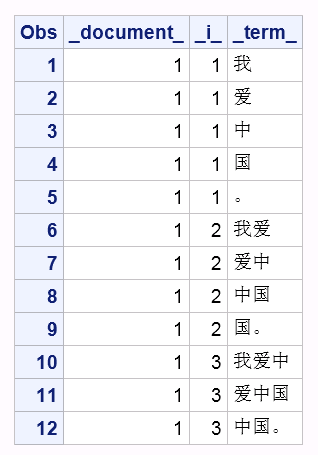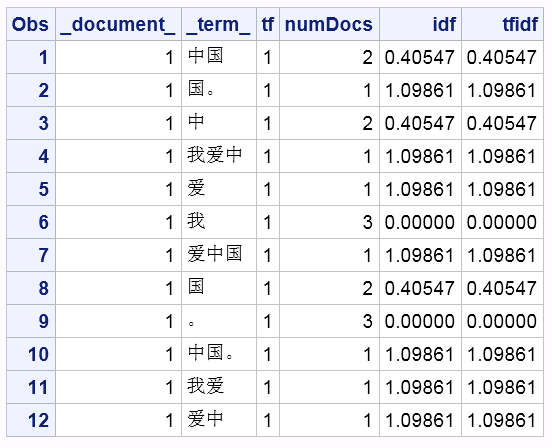SAS Visual Text Analytics provides dictionary-based and non-domain-specific tokenization functionality for Chinese documents, however sometimes you still want to get N-gram tokens. This can be especially helpful when the documents are domain-specific and most of the tokens are not included into the SAS-provided Chinese dictionary.

What is an N-gram?

An N-gram is a sequence of N items from a given text with n representing any positive integer starting from 1. When n is 1, it refers to a unigram; when n is 2, it refers to a bigram; when n is 3, it refers to a trigram. For example, suppose we have a text in Chinese "我爱中国。", which means "I love China." Its N-gram sequence looks like the following:

 n Size N-gram Sequence 1 [我], [爱], [中], [国], [。] 2 [我爱], [爱中], [中国], [国。] 3 [我爱中], [爱中国], [中国。]

How many N-gram tokens are in a given sentence?

If Token_Count_of_Sentence is number of words in a given sentence, then the number of N-grams would be:

Count of N-grams = Token_Count_of_Sentence – ( n - 1 )

The following table shows the N-gram token count of "我爱中国。" with different n sizes.

 n Size N-gram Sequence Token Count 1 [我], [爱], [中], [国], [。] 5 = 5- (1-1) 2 [我爱], [爱中], [中国], [国。] 4 = 5- (2-1) 3 [我爱中], [爱中国], [中国。] 3 = 5- (3-1)

In real actual language processing (NLP) tasks, we often want to get unigram, bigram and trigram together when we set N as 3. Similarly, when we set N as 4, we want to get unigram, bigram, trigram, and four-gram together.

N-gram theory is very simple and under some conditions it has big advantage over dictionary-based tokenization method, especially when the corpus you are working on has many vocabularies out of the dictionary or you don't have a dictionary at all.

How to get N-grams with SAS?

SAS is a powerful programming language when you manipulate data. Below you'll find a program I wrote, using the DATA step to get N-grams.

 data data_test; infile cards dlm='|' missover; input _document_ text :\$100.; cards; 1|我爱中国。 ; run;   data NGRAMS; set data_test; _tmpStr_ = text; do while (klength(_tmpStr_)>0); _maxN_=min(klength(_tmpStr_), 3); do _i_=1 to _maxN_; _term_ = ksubstr(_tmpStr_, 1, _i_); output; end; if klength(_tmpStr_)>1 then _tmpStr_ = ksubstr(_tmpStr_, 2); else _tmpStr_ = ''; end; keep _document_ _term_ _i_; run;

Let's see the SAS results.

 proc sort data=NGRAMS; by _document_ _i_; run;   proc print; run;N-grams tokenization is the first step of NLP tasks. For most NLP tasks the second step is to calculate the term frequency–inverse document frequency (TF-IDF). Here's the approach:

tfidf(t,d,D) = tf(t,d) * idf(t,D)
IDF(t) = log_e(total number of documents / number of documents that contain term t)

Where t denotes the terms; d denotes each document; D denotes the collection of documents.

Suppose that you need to handle process lots of documents -- let me show you how to do it using SAS Viya. I used these four steps.

Step 1: Start CAS Server and create a CAS library.

 cas casauto host="host.example.com" port=5570; libname mycas cas;

Here to simply the code, I only tried 3 sentences for demo purpose. data mycas.data_test; infile cards dlm='|' missover; input _document_ fact :\$100.; cards; 1|我爱中国。 2|我是中国人。 3|我是山西人。 ; run;

Once the data in loaded to CAS, you may run following code to check the column information and record count of your corpus.

 proc cas; table.columnInfo / table="data_test"; run;   table.recordCount / table="data_test"; run; quit;

Step 3: Tokenize texts into N-grams

 %macro TextToNgram(dsin=, docvar=, textvar=, N=, dsout=); proc cas; loadactionset "dataStep"; dscode = "data &dsout; set &dsin; length _term_ varchar(&N); _tmpStr_ = &textvar; do while (klength(_tmpStr_)>0); _maxN_=min(klength(_tmpStr_), &N); do _i_=1 to _maxN_; _term_ = ksubstr(_tmpStr_, 1, _i_); output; end; if klength(_tmpStr_)>1 then _tmpStr_ = ksubstr(_tmpStr_, 2); else _tmpStr_ = ''; end; keep &docvar _term_; run;"; runCode code = dscode; run; quit; %mend TextToNgram;   %TextToNgram(dsin=data_test, docvar=_document_, textvar=text, N=3, dsout=NGRAMS);

Step 4: Calculate TF-IDF.

 %macro NgramTfidfCount(dsin=, docvar=, termvar=, dsout=); proc cas; simple.groupBy / table={name="&dsin"} inputs={"&docvar", "&termvar"} aggregator="n" casout={name="NGRAMS_Count", replace=true}; run; quit;   proc cas; simple.groupBy / table={name="&dsin"} inputs={"&docvar", "&termvar"} casout={name="term_doc_nodup", replace=true}; run;   simple.groupBy / table={name="term_doc_nodup"} inputs={"&docvar"} casout={name="doc_nodup", replace=true}; run; numRows result=r/ table={name="doc_nodup"}; totalDocs = r.numrows; run;   simple.groupBy / table={name="term_doc_nodup"} inputs={"&termvar"} aggregator="n" casout={name="term_numdocs", replace=true}; run;   mergePgm = "data &dsout;" || "merge NGRAMS_Count(keep=&docvar &termvar _score_ rename=(_score_=tf)) term_numdocs(keep=&termvar _score_ rename=(_score_=numDocs));" || "by &termvar;" || "idf=log("||totalDocs||"/numDocs);" || "tfidf=tf*idf;" || "run;"; print mergePgm; dataStep.runCode / code=mergePgm; run; quit; %mend NgramTfidfCount;   %NgramTfidfCount(dsin=NGRAMS, docvar=_document_, termvar=_term_, dsout=NGRAMS_TFIDF);

Now let's see the TFIDF result of the first sentence.

 proc print data=sascas1.NGRAMS_TFIDF; where _document_=1; run;These N-gram methods are not designed only for Chinese documents; and documents in any language can be tokenized with this method. However, the tokenization granularity of English documents is different from Chinese documents, which is word-based rather than character-based. To handle English documents, you only need to make small changes to my code.

How to get N-grams and TF-IDF count from Chinese documents was published on SAS Users.